# Intermediate Algebra Tutorial 23

Intermediate Algebra
Tutorial 23: Exponents and Scientific Notation, Part I

WTAMU > Virtual Math Lab > Intermediate AlgebraLearning Objectives

After completing this tutorial, you should be able to:
1. Use the definition of exponents.
2. Simplify exponential expressions involving multiplying like bases, zero as an exponent, dividing like bases, and negative exponents.
3. Write a number in scientific notation.
4. Write a number in standard notation, without exponents.Introduction

This tutorial covers the basic definition and some of the rules of exponents.  The rules it covers are the product rule and quotient rule, as well as the definitions for zero and negative exponents. Exponents are everywhere in algebra and beyond.  We will also dabble in looking at the basic definition of scientific notation, an application that involves writing the number using an exponent on 10.  Let's see what we can do with exponents.Tutorial

Definition of Exponents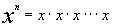(note there are x's in the product)

x = base,    n = exponent

Exponents are another way to write multiplication.

The exponent tells you how many times a base appears in a PRODUCT.Example 1: Evaluate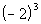.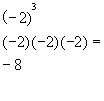*Write the base -2 in a product 3 times
*MultiplyExample 2: Evaluate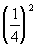.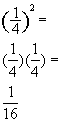*Write the base 1/4 in a product 2 times

*Multiply

Multiplying Like Bases With Exponents
(The Product Rule for Exponents)

Specific Illustration

Let’s first start by using the definition of exponents to help you to understand how we get to the law for multiplying like bases with exponents: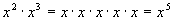Note that 2 + 3 = 5, which is the exponent we ended up with.  We had 2 x’s written in a product plus another 3 x’s written in the product for a total of 5 x’s in the product.  To indicate that we put the 5 in the exponent.

Let's put this idea together into a general rule:

Multiplying Like Bases With Exponents
(The Product Rule for Exponents)

in general,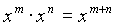In other words, when you multiply like bases you add your exponents

The reason is, exponents count how many of your base you have in a product, so if you are continuing that product, you are adding on to the exponents.Example 3:   Use the product rule to simplify the expression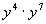.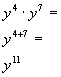Example 4:   Use the product rule to simplify the expression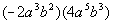.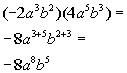Zero as an ExponentExcept for 0, any base raised to the 0 power simplifies to be the number 1.

Note that the exponent doesn’t become 1, but the whole expression simplifies to be the number 1.Example 5:  Evaluate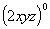.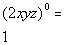*Any expression raised to the 0 power simplifies to be 1Example 6:  Evaluate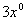.

Be careful on this example.  As shown in Tutorial 4: Operations on Real Numbers, the order of operations says to evaluate exponents before doing any multiplication.  This means we need to find x raised to the 0 power first and then multiply it by 3.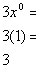*x raised to the 0 power is 1
*Multiply

Dividing Like Bases With Exponents
(Quotient Rule for Exponents)

Specific Illustration

Let’s first start by using the definition of exponents to help you to understand how we get to the law for dividing like bases with exponents: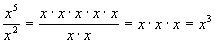Note how 5 - 2 = 3, the final answer’s exponent.  When you multiply you are adding on to your exponent, so it should stand to reason that when you divide like bases you are taking away from your exponent.

Let's put this idea together into a general rule:

Dividing Like Bases With Exponents
(Quotient Rule for Exponents)

in general,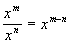In other words, when you divide like bases you subtract their exponents.

Keep in mind that you always take the numerator’s exponent minus your denominator’s exponent, NOT the other way around.Example 7:  Find the quotient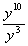.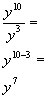*When div. like bases you subtract your exponentsExample 8:  Find the quotient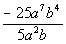.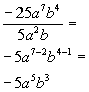*When div. like bases you subtract your exponents

Negative Exponents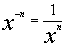or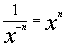Be careful with negative exponents.  The temptation is to negate the base, which would not be a correct thing to do. Since exponents are another way to write multiplication and the negative is in the exponent, to write it as a positive exponent we do the multiplicative inverse which is to take the reciprocal of the base.Example 9:  Simplify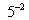.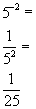*Rewrite with a pos. exp. by taking recip. of base

*Use def. of exponents to evaluateExample 10:  Simplify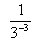.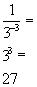*Rewrite with a pos. exp. by taking recip. of base

*Use def. of exponents to evaluate

Simplifying an Exponential Expression

When simplifying an exponential expression,  write it so that each base is written one time with one POSITIVE exponent

In other words, write it in the most condense form you can making sure that all your exponents are positive.

A lot of times you are having to use more than one rule to get the job done.  As long as you are using the rule appropriately, you should be fine.Example 11:    Simplify.  Write answer with positive exponents.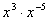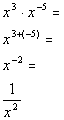Example 12:    Simplify.  Write answer with positive exponents.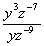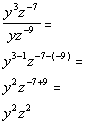Example 13:    Simplify.  Write answer with positive exponents.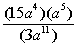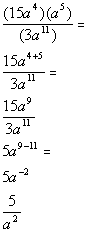Be careful going into the last line.  Note that you do not see an exponent written with the number 5.  This means that the exponent on 5  is understood to be 1.  Since it doesn't have a negative exponent, we DO NOT take the reciprocal of 5.  The only base that has a negative exponent is a, so a is the only base we take the reciprocal of.

Scientific Notation

A positive number is written in scientific notation if it is written in the form: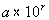where 1 < a < 10 and r is an integer power of 10.

Writing a Number in Scientific Notation

Step 1:  Move the decimal point so that you have a number that is between 1 and 10.

In other words, you will put your decimal after the first non zero number.

Step 2:   Count the number of decimal places moved in Step 1 .

If the decimal point was moved to the left, the count is positive.

If the decimal point is moved to the right, the count is negative.

Step 3:   Write as a product of the number (found in Step 1) and 10 raised to the power of the count (found in Step 2).Example 14:    Write the number in scientific notation:   483,000,000.

Step 1:  Move the decimal point so that you have a number that is between 1 and 10.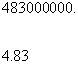*Decimal is at the end of the number

*Move decimal to create a number between 1 and 10

Step 2:   Count the number of decimal places moved in Step 1 .

How many decimal places did we end up moving?
We started at the end of the number 483000000 and moved it between the 4 and 8.  That looks like a move of 8 places.

What direction did it move?
Looks like we moved it to the left.

So, our count is +8.

Step 3:   Write as a product of the number (found in Step 1) and 10 raised to the power of the count (found in Step 2).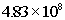Note how the number we started with is a bigger number than the one we are multiplying by in the scientific notation.  When that is the case, we will end up with a positive exponentExample 15:    Write the number in scientific notation:   .00054.

Step 1:  Move the decimal point so that you have a number that is between 1 and 10.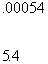*Decimal is at the beginning of the number

*Move decimal to create a number between 1 and 10

Step 2:   Count the number of decimal places moved in Step 1 .

How many decimal places did we end up moving?
We started at the beginning of the number .00054  moved it between the 5 and 4.  That looks like a move of 4 places.

What direction did it move?
Looks like we moved it to the right.

So, our count is - 4.

Step 3:   Write as a product of the number (found in Step 1) and 10 raised to the power of the count (found in Step 2).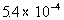Note how the number we started with is a smaller number than the one we are multiplying by in the scientific notation.  When that is the case we will end up with a negative exponent.

Write a Scientific Number in Standard Form

Basically, you just multiply the first number times the power of 10.

Whenever you multiply by a power of 10, in essence what you are doing is moving your decimal place.

If the power on 10 is positive, you move the decimal place that many units to the right.

If the power on 10 is negative, you move the decimal place that many units to the left.

Make sure you add in any zeros that are neededExample 16:  Write the number in standard notation, without exponents.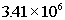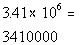*Move the decimal 6 to the rightExample 17:  Write the number in standard notation, without exponents.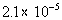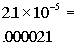*Move the decimal 5 to the leftPractice Problems

These are practice problems to help bring you to the next level.  It will allow you to check and see if you have an understanding of these types of problems. Math works just like anything else, if you want to get good at it, then you need to practice it.  Even the best athletes and musicians had help along the way and lots of practice, practice, practice, to get good at their sport or instrument.  In fact there is no such thing as too much practice.

To get the most out of these, you should work the problem out on your own and then check your answer by clicking on the link for the answer/discussion for that  problem.  At the link you will find the answer as well as any steps that went into finding that answer.Practice Problem 1a - 1d: Simplify.

1a.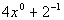1b.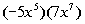1c.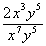1d.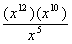Practice Problem 2a: Write the number in scientific notation.

2a.      .00000146Practice Problem 3a: Write the number in standard notation, without exponents.

3a.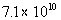Need Extra Help on these Topics?

The following are webpages that can assist you in the topics that were covered on this page:

http://www.sosmath.com/algebra/logs/log2/log2.html#shortcuts
This webpage helps you with the definition of exponents.

http://www.sosmath.com/algebra/logs/log3/log31/log31.html
This webpage helps with the product rule for exponents.

http://www.sosmath.com/algebra/logs/log3/log32/log32.html
This webpage helps with the quotient rule for exponents.

Go to Get Help Outside the Classroom found in Tutorial 1: How to Succeed in a Math Class for some more suggestions.Last revised on July 11, 2011 by Kim Seward.